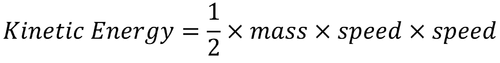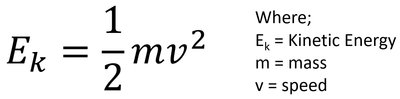# Kinetic Energy Store

## Key Stage 3

### Meaning

The kinetic energy store is the energy store associated with moving objects.

### About The Kinetic Energy Store

The faster an object moves the more energy it has in its kinetic store.
If two objects are moving at the same speed the one with more mass has more energy in the kinetic store.

### EquationThe equation for kinetic energy written in words.The equation for kinetic energy written in symbols.

### Calculating Kinetic Energy

 A 700kg formula one racing car has a top speed of 100m/s. Calculate the kinetic energy of the car to two significant figures. A cheetah of mass 75kg runs at a speed of 32m/s. Calculate the kinetic energy of the cheetah correct to two significant figures. A 160g cricket ball is hit at 44m/s. Calculate the kinetic energy of the cricket ball correct to two significant figures. 1. State the known quantities m = 700kg v = 100m/s 1. State the known quantities m = 75kg v = 32m/s 1. State the known quantities m = 160g Convert mass into kilograms. min kilograms = $$\frac{160}{1000}$$ m = 0.16kg v = 32m/s 2. Substitute the numbers into the equation and solve. $$E_k = \frac{1}{2} m v^2$$ $$E_k = \frac{1}{2} \times 700 \times 100^2$$ $$E_k = \frac{1}{2} \times 700 \times 10,000$$ $$E_k = 3,500,000J$$ 2. Substitute the numbers into the equation and solve. $$E_k = \frac{1}{2} m v^2$$ $$E_k = \frac{1}{2} \times 75 \times 32^2$$ $$E_k = \frac{1}{2} \times 75 \times 1024$$ $$E_k = 38,400J$$ $$E_k \approx 38000J$$ 2. Substitute the numbers into the equation and solve. $$E_k = \frac{1}{2} m v^2$$ $$E_k = \frac{1}{2} \times m \times v^2$$ $$E_k = \frac{1}{2} \times 0.16 \times 44^2$$ $$E_k = \frac{1}{2} \times 0.16 \times 1936$$ $$E_k = 154.88J$$ $$E_k \approx 150J$$

## Key Stage 4

### Meaning

The kinetic energy store is the energy store associated with moving objects.

Any moving object stores kinetic energy.
The kinetic energy store of an object is related to two properties of the object:

### Equation

NB: You must memorise this equation!

Kinetic Energy = 0.5 x (Mass) x (Speed)2

$$E_k = \frac{1}{2} m v^2$$

Where:

Ek = Kinetic Energy stored.

m = The mass of the object.

v = The speed or velocity of the object.

### Calculating Kinetic Energy

 A 700kg formula one racing car has a top speed of 100m/s. Calculate the kinetic energy of the car to two significant figures. A cheetah of mass 75kg runs at a speed of 32m/s. Calculate the kinetic energy of the cheetah correct to two significant figures. A 160g cricket ball is hit at 44m/s. Calculate the kinetic energy of the cricket ball correct to two significant figures. 1. State the known quantities m = 700kg v = 100m/s 1. State the known quantities m = 75kg v = 32m/s 1. State the known quantities m = 160g Convert mass into kilograms. min kilograms = $$\frac{160}{1000}$$ m = 0.16kg v = 32m/s 2. Substitute the numbers into the equation and solve. $$E_k = \frac{1}{2} m v^2$$ $$E_k = \frac{1}{2} \times 700 \times 100^2$$ $$E_k = \frac{1}{2} \times 700 \times 10,000$$ $$E_k = 3,500,000J$$ 2. Substitute the numbers into the equation and solve. $$E_k = \frac{1}{2} m v^2$$ $$E_k = \frac{1}{2} \times 75 \times 32^2$$ $$E_k = \frac{1}{2} \times 75 \times 1024$$ $$E_k = 38,400J$$ $$E_k \approx 38000J$$ 2. Substitute the numbers into the equation and solve. $$E_k = \frac{1}{2} m v^2$$ $$E_k = \frac{1}{2} \times m \times v^2$$ $$E_k = \frac{1}{2} \times 0.16 \times 44^2$$ $$E_k = \frac{1}{2} \times 0.16 \times 1936$$ $$E_k = 154.88J$$ $$E_k \approx 150J$$

### Calculating Velocity from Kinetic Energy

 A 75kg person has a kinetic energy of 96J while while walking. Calculate their speed. A bird of mass 2.4kg flies at a constant speed with a kinetic energy store of 1500J. Calculate the speed of the bird correct to two significant figures. A 60g bullet has a kinetic energy store of 750J. Calculate the speed of the bullet correct to two significant figures. 1. State the known quantities Ek = 96J m = 75kg 1. State the known quantities Ek = 1500J m = 2.4kg 1. State the known quantities Ek = 750J m = 60g = 0.06kg 2. Substitute the numbers and evaluate. $$E_k = \frac{1}{2} m v^2$$ $$96 = \frac{1}{2} \times 75 \times v^2$$ $$96 = 37.5v^2$$ 2. Substitute the numbers and evaluate. $$E_k = \frac{1}{2} m v^2$$ $$1500 = \frac{1}{2} \times 2.4 \times v^2$$ $$1500 = 1.2v^2$$ 2. Substitute the numbers and evaluate. $$E_k = \frac{1}{2} m v^2$$ $$750 = \frac{1}{2} \times 0.06 \times v^2$$ $$750 = 0.03v^2$$ 3. Rearrange the equation and solve. $$v^2 = \frac{96}{37.5}$$ $$v = \sqrt{\frac{96}{37.5}}$$ $$v = 1.6m/s$$ 3. Rearrange the equation and solve. $$v^2 = \frac{1500}{1.2}$$ $$v = \sqrt{\frac{1500}{1.2}}$$ $$v = 35.35534m/s$$ $$v \approx 35m/s$$ 3. Rearrange the equation and solve. $$v^2 = \frac{750}{0.06}$$ $$v = \sqrt{\frac{750}{0.06}}$$ $$v = 158.1139m/s$$ $$v \approx 160m/s$$